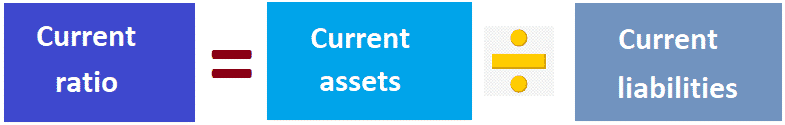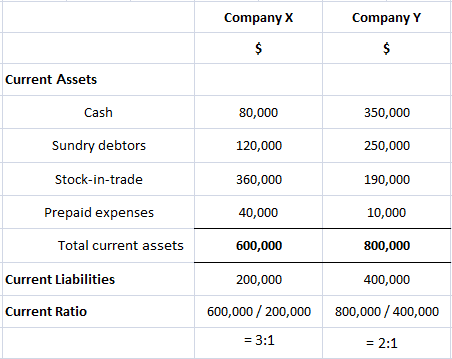## Current Ratio: Definition

The current ratio or working capital ratio is a ratio of current assets to current liabilities within a business. In other words, it is defined as the total current assets divided by the total current liabilities.

The current ratio is one of the oldest ratios used in liquidity analysis. It measures the number of times that the current liabilities can be paid using the company’s available current assets.

## Current Ratio: Explanation

The current ratio relates the current assets of the business to its current liabilities. This ratio was designed to assist decision-makers when determining a firm’s ability to pay its current liabilities from its current assets.

The current assets are cash or assets that are expected to turn into cash within the current year. On the other hand, the current liabilities are those that must be paid within the current year.

## Formula For Current Ratio

The current ratio is calculated using the formula shown below.The numerator generally includes assets such as cash, short-term marketable securities, sundry debtors (accounts receivables), stocks (inventories), and prepaid expenses.

The denominator, on the other hand, includes sundry creditors (Accounts Payables), dividends, taxes due, and short-term bank loans.

A 2:1 current ratio is generally considered satisfactory by creditors. However, they should not rely too heavily on the current ratio.

This is because it is possible to manipulate a company’s current assets at the end of the accounting period to produce a ratio that may satisfy creditors, while in reality, it weakens the immediate liquidity position of the company.

For instance, the liquidity positions of companies X and Y are shown below.Company X has a better current ratio compared to Company Y.

However, an examination of the composition of current assets reveals that the total cash and debtors of Company X account for merely one-third of the total current assets.

By contrast, in the case of Company Y, 75% of the current assets are made up of these two liquid resources.

Hence, Company Y’s ability to meet its current obligations can in no way be considered worse than X’s.

It’s important to note here that Company X can further improve its current ratio by a slight manipulation (i.e., if it uses the \$80,000 cash in hand to pay off current liabilities, the current ratio can to that extent be improved further).

The new ratio would be:The current ratio is a rough indicator of the degree of safety with which short-term credit may be extended to the business.

Generally, the assumption is made that the higher the current ratio, the better the creditors’ position due to the higher probability that debts will be paid when due.

A high current ratio is not beneficial to the interest of shareholders. This is because it could mean that the company maintains an excessive cash balance or has over-invested in receivables and inventories.

Even from the point of view of creditors, a high current ratio is not necessarily a safeguard against non-payment of debts.

This is because a high current ratio represents two things: either an excess of cash or its equivalent relative to the current needs or a preponderance of current assets having low liquidity.

Therefore, it is necessary for an analyst, in addition to taking note of any window dressing a company may have engaged in, to consider the following before drawing any conclusions:

1. Distribution of current assets
2. Types of business (manufacturing or retail?)
3. Terms granted by creditors to company and by company to customers

## Expressing the Current Ratio

The current ratio can be expressed in any of the following three ways, but the most popular approach is to express it as a number. An overview of the three approaches is given below.

### Expressed as a Proportion

The first way to express the current ratio is to express it as a proportion (i.e., current liabilities to current assets). In this case, current liabilities are expressed as 1 and current assets are expressed as whatever proportionate figure they come to.

For example, if current liabilities are \$40,000 and current assets are \$60,000, the current ratio would be: \$40,000 : \$60,000, or 1 : 1.5.

### Expressed as a Number

This is arrived at by dividing current assets by current liabilities.

For example, if a company’s total current assets are \$90,000 and its current liabilities are \$72,000, its current ratio is \$90,000/\$72,000 = 1.25.

If the current ratio of a business is 1 or more, it means it has more current assets than current liabilities (i.e., positive working capital).

However, if the current ratio of a company is below 1, it shows that it has more current liabilities than current assets (i.e., negative working capital). Such a company is said to be an over-trading company.

Over-trading companies are likely to face substantial difficulties in meeting their day-to-day obligations.

### Expressed as a Percentage

The current ratio expressed as a percentage is arrived at by showing the current assets of a company as a percentage of its current liabilities.

For example, if a company’s current assets are \$80,000 and its current liabilities are \$64,000, its current ratio is 125%.

If a company has a current ratio of 100% or above, this means that it has positive working capital. A current ratio of less than 100% indicates negative working capital.

## Example

The current ratio for Sample Limited is calculated as follows:

Current Ratio = 490,000 / 185,000 = 2.65:1

As shown above, the company’s current ratio is 2.65: 1. In other words, for every dollar of current liabilities, there is \$2.65 in current assets. So, a ratio of 2.65 means that Sample Limited has more than enough cash to meet its immediate obligations.

At one time, there was an old rule of thumb that the current ratio should be at least 2:1 to provide the proper margin of safety. However, some industries may require more or less.

In actual practice, the current ratio tends to vary by the type and nature of the business. Everything is relative in the financial world, and there are no absolute norms.

### What is a current ratio?

The current ratio is an evaluation of a company's short-term liquidity. In simplest terms, it measures the amount of cash available relative to its liabilities. Higher ratios indicate more liquidity and greater solvency.

### How do you calculate the current ratio?

There are several methods for calculating this ratio. Companies can divide the total value of its current assets by the total value of its current liabilities, or they can take a division of their current assets and dividing it by their average current liabilities over a period.

### What do the results mean?

The higher the number, the more liquid and/or stable a company. A low current ratio may indicate the company is not able to cover its current liabilities without having to sell its investments or delay payment on its own debts.

### What does a high/low current ratio mean?

The prevailing view of what constitutes a "good" ratio has been changing in recent years, as more companies have looked to the future rather than just the current moment. Some lenders and investors have been looking for a 2-3 ratio, while others have said 1 to 1 is good enough. It all depends on what you're trying to achieve as a business owner or investor.

### How does Working Capital relate to liquidity?

Working Capital is the difference between current assets and current liabilities. A business' liquidity is determined by the level of cash, marketable securities, Accounts Receivable, and other liquid assets that are easily converted into cash. The more liquid a company's balance sheet is, the greater its Working Capital (and therefore its ability to maneuver in times of crisis).

True is a Certified Educator in Personal Finance (CEPF®), a member of the Society for Advancing Business Editing and Writing, contributes to his financial education site, Finance Strategists, and has spoken to various financial communities such as the CFA Institute, as well as university students like his Alma mater, Biola University, where he received a bachelor of science in business and data analytics.

To learn more about True, visit his personal website, view his author profile on Amazon, his interview on CBS, or check out his speaker profile on the CFA Institute website.